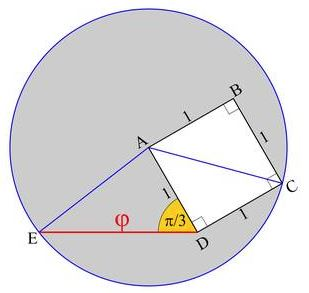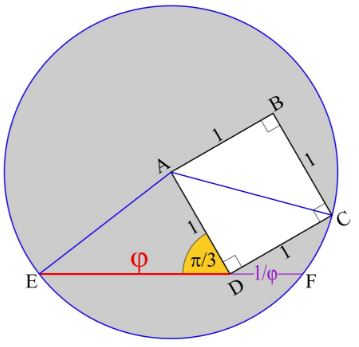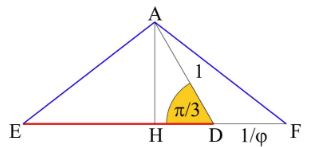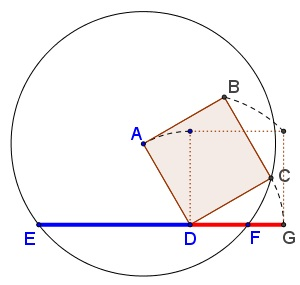# Rotating Square in Search of the Golden Ratio

### John Arioni 18 March, 2017

Draw square $ABCD\,$ with sides equal to $1\,$ (side $AB\,$ horizontal) and rotate it counterclockwise around vertex $D\,$ through angle $\displaystyle \frac{\pi}{6}.\,$ Then draw a circle $A(C)\,$ with cener $A\,$ through $C.\,$ Obviously, the radius of the circle equals $\sqrt{2}.\,$ Let $E\,$ be on the circle, making $DE\,$ horizontal, as in the diagran below:Note that $\angle ADE=\displaystyle \frac{\pi}{3}.\,$ Apply the Law of Cosines in $\Delta ADE:$

$\displaystyle AE^2=AD^2+DE^2-2AD\cdot DE\cos\frac{\pi}{3},$

so, letting x=DE, we have

$\displaystyle x^2-x+(1-2)=x^2-x-1=0.$

Taking the positive root we find that $x=\varphi,\,$ the Golden Ratio. Let $F\,$ be the second intersection of $DE\,$ with the circle:We shall prove that $DF=\displaystyle \frac{1}{\varphi}.\,$ To this end consider the simplified diagram:We have $AH=\sin\displaystyle \frac{\pi}{3}=\frac{3}{2},\,$ so that $HF=\sqrt{AF^2-AH^2}=\displaystyle \frac{\sqrt{5}}{2};\,$ $HD=\cos\displaystyle \frac{\pi}{3}=\frac{1}{2},\,$ and, finally, $DF=HF-HD=\displaystyle \frac{\sqrt{5}}{2}-\frac{1}{2}=\frac{1}{\varphi}.$

Obviously, if $G\,$ is the former (before the rotation) position of point $C\,$ then $\displaystyle \frac{EG}{ED}=\frac{ED}{DG}=\varphi:$### Golden Ratio